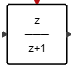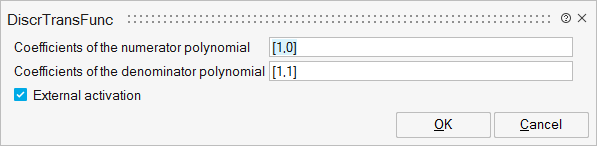# DiscrTransFunc

This block implements, when activated at a fixed rate, a linear system represented by its rational transfer function. The transfer function must be proper, meaning that the degree of the numerator must not exceed that of the denominator. In the MIMO case, the numerator and denominator must be defined as cells (see OML tf2ssc function for details).Dynamical

## Description

This block implements, when activated at a fixed rate, a linear system represented by its rational transfer function. The transfer function must be proper, meaning that the degree of the numerator must not exceed that of the denominator. In the SISO case, the input and output may be of arbitrary but equal size, in which case the SISO system is applied element-wise.

## ParametersNameLabelDescriptionData TypeValid Values

num

Coefficients of the numerator polynomial

This parameter defines the numerator of the transfer function, which is a polynomial in z domain. The entries of the vector are the coefficients of this polynomial. Coefficients should be given in a m-by-n matrix where m is the number of output and n is the order of the numerator polynomial. A cell of vectors is used in the MIMO case.

den

Coefficients of the denominator polynomial

This parameter defines the denominator of the transfer function, which is a polynomial in z domain. The entries of the vector are the coefficients of this polynomial. A cell of vectors is used in the MIMO case.

externalActivation

External activation

This parameter defines if the block receives an external activation or inherits its activation through its regular input ports. When External Activation is used, an additional activation port is added to the block.

Number

0
1

## Ports

NameTypeDescriptionIO TypeNumber

Port 1

explicit

output

1

Port 2

explicit

input

1

Port 3

activation

input

externalActivation

NameValueDescription

always active

no

Standard mode activated

direct-feedthrough

no

no, unless the order of numerator is equal to the order of denominator.

zero-crossing

no

mode

no

continuous-time state

no

discrete-time state

yes